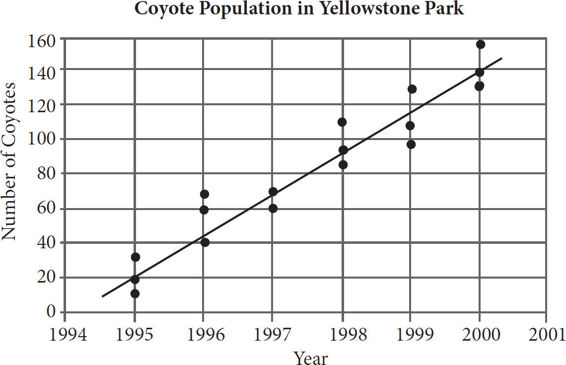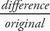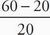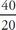# SAT Math Multiple Choice Question 89: Answer and Explanation

### Test Information

Question: 89

14. In the 1990s, the park rangers at Yellowstone National Park implemented a program aimed at increasing the dwindling coyote population in Montana. Results of studies of the coyote population in the park are shown in the scatterplot below.According to the data in the scatterplot, which of the following best represents the percent increase between the median of the results of the studies from 1995 and the median of the results of the studies from 1996 ?

• A. 50%
• B. 100%
• C. 150%
• D. 200%

D The median number of coyotes in the park in 1995 was 20, and the median number of coyotes in the park in 1996 was 60. (Be careful to RTFQ: the question wants median, NOT line of best fit!) In order to calculate the percent increase, it is necessary to use the percent change formula:× 100. The calculation here will be× 100 =× 100 = 2 × 100 = 200%, which is (D).